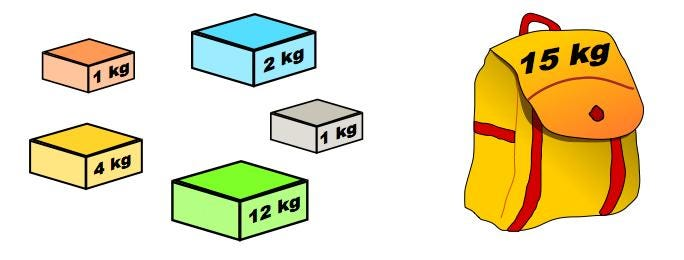# 0 - 1 Knapsack Problem

05/13/20192 Min Read — In Algorithm

Let's discuss one of my favorite DP problem.Given weights and values of n items, put these items in a knapsack of capacity W to get the maximum total value in the knapsack. In other words, given two integer arrays val[0..n-1] and wt[0..n-1] which represent values and weights associated with n items respectively. Also given an integer W which represents knapsack capacity, find out the maximum value subset of val[] such that sum of the weights of this subset is smaller than or equal to W. You cannot break an item, either pick the complete item, or don’t pick it (0-1 property).

### Recursive Solution

#### Idea

Let's see if we can find the subproblem so that this problem can be solved recursively.

1. Looking at the last item; it could be included or excluded in the optimal solution.
2. Taking out item one by one until we run out capacity
3. When the capacity is zero or knapsack is empty, the max value is 0

#### Implementation

Code the rules listed above in Python

``````'''
@W:int -> capacity
@wt:int[] -> array of item's weights
@val:int[] -> array of item's values
@n:int[] -> number of items
'''
def knapSackRec(W, wt, val, n):
if W == 0 or n == 0:
return 0
lastWeight = wt[n - 1]
lastValue = val[n - 1]
if (W - lastWeight < 0):
return knapSackRec(W, wt, val, n - 1) # last item will not be included
return max(
lastValue + knapSackRec(W - lastWeight, wt, val, n - 1),
knapSackRec(W, wt, val, n - 1)
)``````

#### Complexity

To analyze the time complexity, let's begin with very simple case.
Assuming W = 2, n = 3, wi = [1, 1, 1]. Then we can expand subproblems below:

``````                        K(3, 2)  ---------> K(n, W)
/            \
/                \
K(2,2)                  K(2,1)
/       \                  /    \
/           \              /        \
K(1,2)      K(1,1)        K(1,1)     K(1,0)
/  \         /   \          /   \
/      \     /       \      /       \
K(0,2)  K(0,1)  K(0,1)  K(0,0)  K(0,1)   K(0,0)``````

Time: O(2 ^ n)

### DP Solution

#### Idea

Speaking of algorithm part, we will use the idea inspired by recursive solution.
Meanwhile, we need to cache the solution for each subproblem.

#### Implementation

``````'''
@W:int -> capacity
@wt:int[] -> array of item's weights
@val:int[] -> array of item's values
@n:int[] -> number of items
'''
def knapSackDP(W, wt, val, n):
cache = [ * (W + 1) for x in range(n + 1)]
for i in range(n + 1):
for wi in range(W + 1):
if i == 0 or wi == 0:
cache[i][wi] = 0
break

lastWeight = wt[i - 1]
lastValue = val[i - 1]
if (wi - lastWeight) < 0:
cache[i][wi] = cache[i - 1][wi]
else:
cache[i][wi] = max(
lastValue + cache[i - 1][wi - lastWeight],
cache[i - 1][wi]
)
return cache[n][W]``````

Space: O(n * W)
Time: O(n * W)